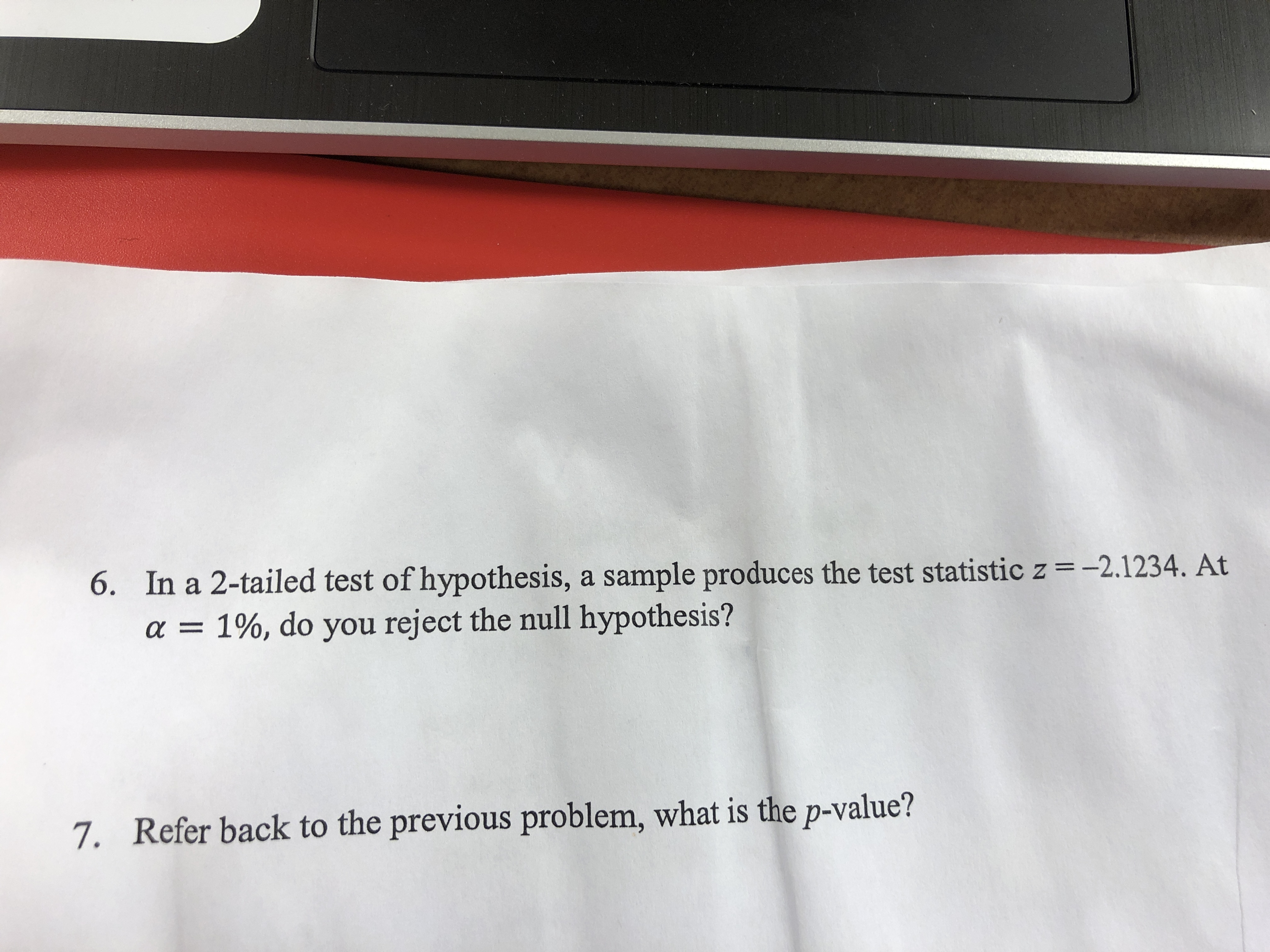# 6.In a 2-tailed test of hypothesis, a sample produces the test statistic z = -2.1234. Ata= 1% , do you reject the null hypothesis?7. Refer back to the previous problem, what is the p-value?

Question
120 views

6 and 7. Please show TI 84 stepshelp_outlineImage Transcriptionclose6. In a 2-tailed test of hypothesis, a sample produces the test statistic z = -2.1234. At a = 1% , do you reject the null hypothesis? 7. Refer back to the previous problem, what is the p-value? fullscreen
check_circle

Step 1

6.

Step by step procedure to find the p-value using TI-83 is given below:

• Find the value of z.
• Find the absolute value of z.
• Press 2nd DISTR
• Select normalcdf(
• Enter
• Type (2.1234, 1000, 0,1)
• Ent...

### Want to see the full answer?

See Solution

#### Want to see this answer and more?

Solutions are written by subject experts who are available 24/7. Questions are typically answered within 1 hour.*

See Solution
*Response times may vary by subject and question.
Tagged in

### Hypothesis Testing